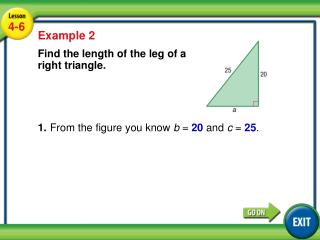DownloadDownload PresentationLesson 4-6 Example 2

# Lesson 4-6 Example 2

Télécharger la présentation## Lesson 4-6 Example 2

- - - - - - - - - - - - - - - - - - - - - - - - - - - E N D - - - - - - - - - - - - - - - - - - - - - - - - - - -
##### Presentation Transcript

1. 4-6 Example 2 Lesson 4-6 Example 2 Find the length of the leg of a right triangle. 1.From the figure you know b = 20 and c = 25.

2. 4-6 Example 2 Lesson 4-6 Example 2 Find the length of the leg of a right triangle. 2. Substitute the values of a and c in the Pythagorean Theorem and solve for b. a2 + b2 = c2 Use the formula. a2 + 202 = 252Substitute the measure of each side.

3. 4-6 Example 2 Lesson 4-6 Example 2 Find the length of the leg of a right triangle. a2 + 400= 625Simplify the exponents. a2 = 225 Subtract 400 from 625. Take the square root of both sides. a = 15 Simplify the square roots.

4. 4-6 Example 2 Lesson 4-6 Example 2 Find the length of the leg of a right triangle. The length of the leg is 15 units.